# Prediction With Expert Advice Under Discounted Loss

The paper describes the application of the idea of Shortcut Defensive Forecasting to compete under discounted loss. It suggests a modification of the Aggregating Algorithm for that. The arXiv technical report contains more detailed description of the idea of the modification, and of the theorems which are possible to prove.

The problem of prediction with expert advice under discounted loss is considered. The cumulative discounted loss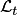is defined as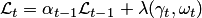for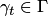,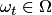, loss function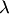(mixable or not), and discounting factors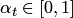. In the case when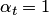, there is no discounting. The case when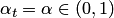is the most popular and is called exponential discounting. The following theorem is the main result of the paper (we state it here only for the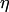-mixable loss functions, but the paper describes the general case). It holds when the number of experts is finite.

Theorem. Learner has a strategy guaranteeing that, for any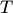and for any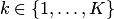, it holds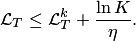The algorithm works as follows.

Initialize weights of experts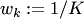,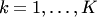.
FORGet discount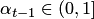.
Get Experts' predictions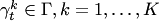.
Calculate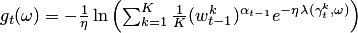, for all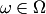.
Output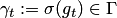.
Get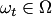.
Update the weights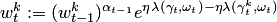,.
ENDFOR

For non-mixable but convex and bounded games, a modification of the Weak Aggregating Algorithm is used to prove an upper bound on the discounted loss of the learner.

Theorem. Suppose thatis a non-empty convex game and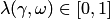for all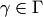and. Learner has a strategy guaranteeing that, for anyand for any, it holds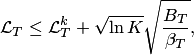where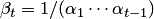and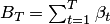.

For the discounted square loss function, it is possible to compete with linear and kernelized linear experts in the online regression framework. Denote by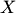the matrix of size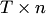consisting of the rows of the input vectors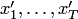. Let also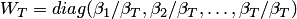, i.e.,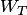is a diagonal matrix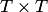.

Theorem. For any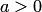, there exists a prediction strategy for Learner in online regression protocol achieving, for everyand for any linear predictor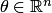,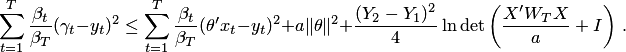If, in addition,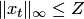for all, then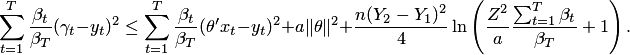Interestingly, instead of the time variable (as in the bound for Aggregating Algorithm Regression), we have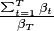. For exponential discountingfor all, we have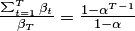.

The method which achieves the bound for linear regression is similar to online weighed least squares.

• Alexey Chernov and Fedor Zhdanov. Prediction with expert advice under discounted loss. In Proceedings of the 21st International Conference on Algorithmic Learning Theory, 2010.